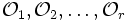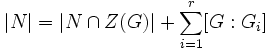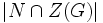Prime power order implies center is normality-large

This article gives the statement and possibly, proof, of an implication relation between two subgroup properties. That is, it states that every subgroup satisfying the first subgroup property (i.e., group of prime power order) must also satisfy the second subgroup property (i.e., group whose center is normality-large)
View all subgroup property implications | View all subgroup property non-implications
Get more facts about group of prime power order|Get more facts about group whose center is normality-large

Statement

Symbolic statement

Let$G$ be a group of prime power order, and$N$ be a normal subgroup. Denote by$Z(G)$ the center of$G$.Then:$N \cap Z(G) = 1 \iff N = 1$

Verbal statement

The center of a group of prime power order is a Normality-large subgroup (?): its intersection with any nontrivial normal subgroup is nontrivial.

Proof

Given: A group$G$ of prime power order with center$Z(G)$, a nontrivial normal subgroup$N$ of$G$.

To prove:$N \cap Z(G)$ is nontrivial.

Proof: Consider the action of$G$ on$N$ by conjugation. This action is well-defined because$N$ is normal in$G$.

The fixed points under this action are the elements of$N$ that commute with every element of$G$ which is$N \cap Z(G)$.

Suppose, under this action, the elements of$N$ that are not fixed points decompose into orbits$\mathcal{O}_1, \mathcal{O}_2, \dots, \mathcal{O}_r$. Then, we have:$|N| = |N \cap Z(G)| + \sum_{i=1}^r |\mathcal{O}_i|$.

By the fundamental theorem of group actions (fact (1)), we have:$|N| = |N \cap Z(G)| + \sum_{i=1}^r [G:G_i]$,

where$G_i$ is the stablizer of some point in$\mathcal{O}_i$.

Since each$\mathcal{O}_i$ has size greater than one,$G_i$ is a proper subgroup, so we have that$[G:G_i] = |G|/|G_i|$ (by fact (3), Lagrange's theorem). Since the order of$G$ is a power of$p$ and$G_i$ is proper,$|G/G_i|$ is a power, and in particular, a multiple of$p$. Thus, we get:$|N| \equiv |N \cap Z(G)| \mod p$

Since$N$ is nontrivial,$|N|$ is a multiple of$p$, hence so is$|N \cap Z(G)|$. Thus,$N \cap Z(G)$ is nontrivial.